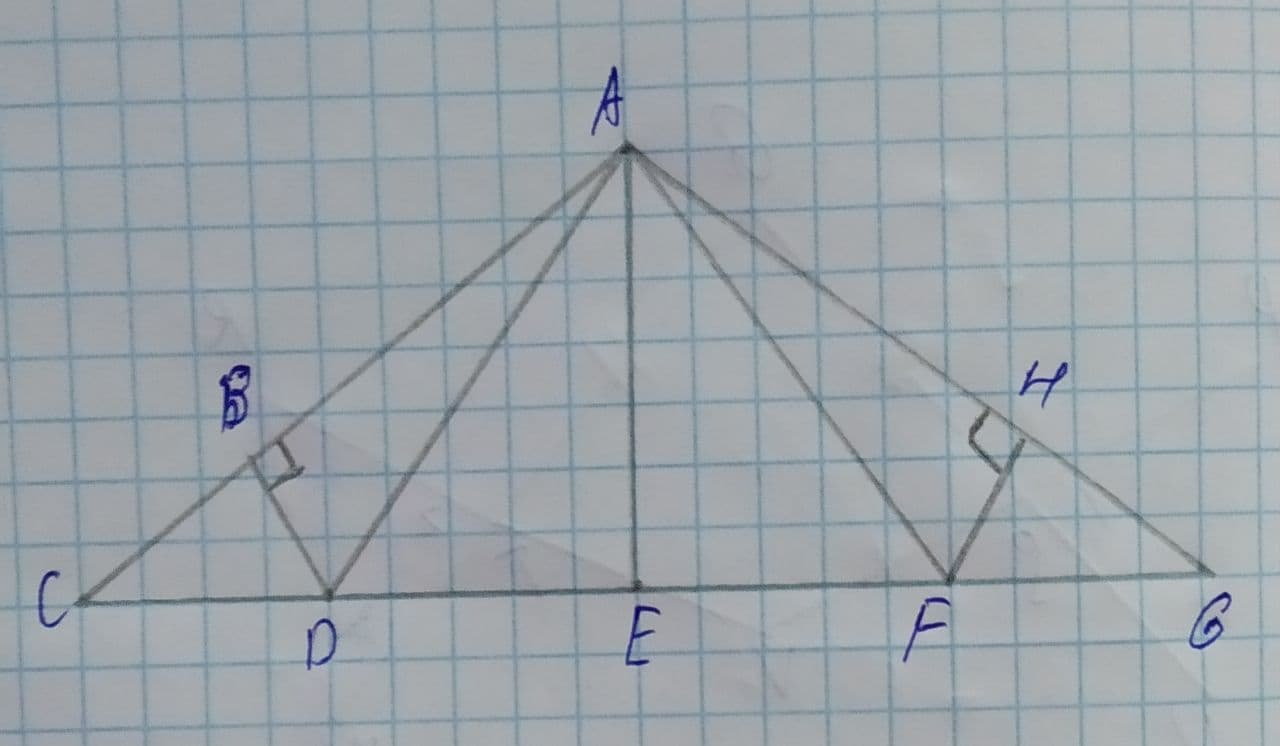# Find AH, in the roof truss shown AB=8 and m\angle HAF=37^{\circ}. The roof truss has line symmetry.alesterp 2021-07-20 Answered
In the roof truss shown, AB=8 and $m\mathrm{\angle }HAF={37}^{\circ }$.
Find: AH
Given:
AB=8 and $m\mathrm{\angle }HAF={37}^{\circ }$.
The roof truss has line symmetry.Figure(1)
You can still ask an expert for help

## Want to know more about Congruence?

• Questions are typically answered in as fast as 30 minutes

Solve your problem for the price of one coffee

• Math expert for every subject
• Pay only if we can solve itClelioo
Approach:
(1) If a figure has line symmetry then one side of the symmetric line is congruent to the other side of the line.
(2) If two triangles are congruent to each other then all sides of the triangle are congruent.
Calculation:
The roof truss has line symmetry about line $over\to \left\{AE\right\}$.
So,
$\mathrm{△}ABD\stackrel{\sim }{=}\mathrm{△}AHF$.
Use transitive property of congruence.
$\mathrm{△}ABD\stackrel{\sim }{=}\mathrm{△}AHF$ and AH, and AB are the sides of congruent triangles.
So,
AH=AB
Substitute 8 for AH in order to find the value of AH.
AH=8
Conclusion:
Therefore, the value of AH is 8.